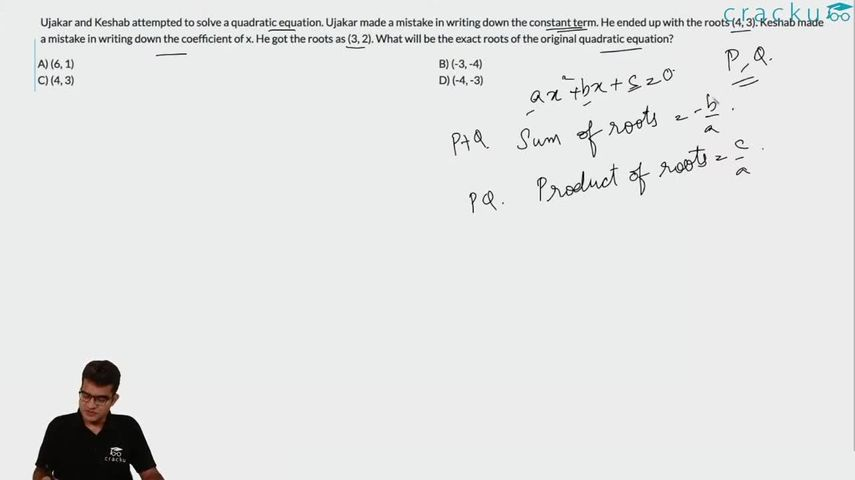Question 47

# Ujakar and Keshab attempted to solve a quadratic equation. Ujakar made a mistake in writing down the constant term. He ended up with the roots (4, 3). Keshab made a mistake in writing down the coefficient of x. He got the roots as (3, 2). What will be the exact roots of the original quadratic equation?

Solution

We know that quadratic equation can be written as $$x^2$$-(sum of roots)*x+(product of the roots)=0.
Ujakar ended up with the roots (4, 3) so the equation is $$x^2$$-(7)*x+(12)=0 where the constant term is wrong.

Keshab got the roots as (3, 2) so the equation is $$x^2$$-(5)*x+(6)=0 where the coefficient of x is wrong .

So the correct equation is $$x^2$$-(7)*x+(6)=0. The roots of above equations are (6,1).

### View Video Solution